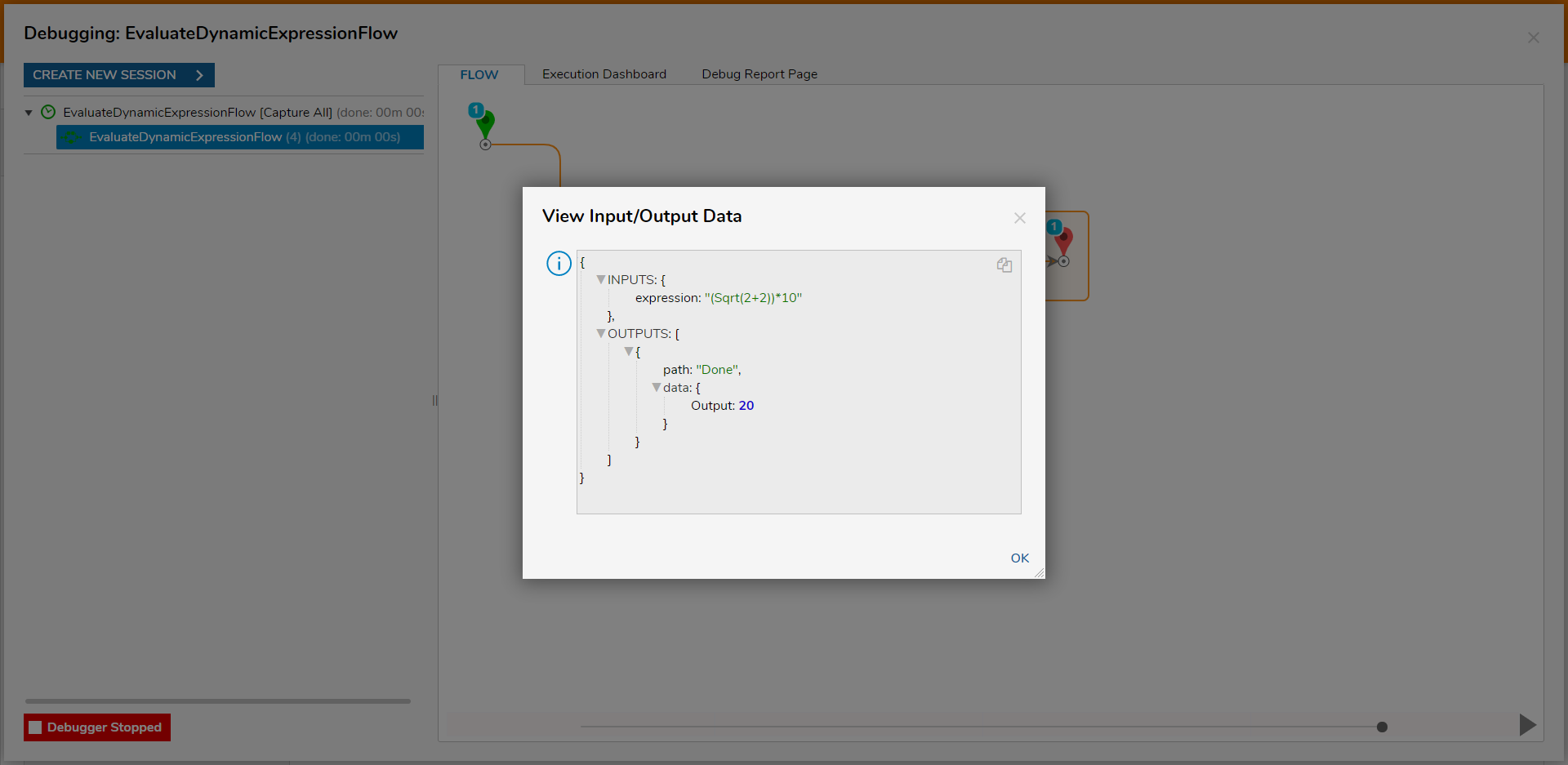• Version 7
Evaluate Expressions
• 26 May 2021
• Dark
Light

# Evaluate Expressions

• Dark
Light

## Overview

There are two expression steps; Evaluate Expression and Evaluate Dynamic Expression. The Evaluate Expression step is used to solve a defined algebraic expression. The step achieves this by automatically creating inputs for characters used to represent the numeric variables in the Expression. This can be helpful for people that work in fields such as banking or engineering, where they may need to evaluate a custom formula with data values from another step. The first example below demonstrates how to utilize an Evaluate Expression step to calculate the average of three test scores; the first exam, the midterm, and the final. The second example demonstrates how to utilize NCalc expressions in conjunction with an Evaluate Dynamic Expression step, to run a calculation.

## Difference in Evaluate Expression and Evaluate Dynamic Expression Steps

The differences between the two Evaluate Expression steps lie in their different input mapping methods; the calculation code is the same for both.
The Evaluate Expression step takes in the Expression solely as a Constant. The Evaluate Dynamic Expression step allows for more dynamic mappings (such as Select From Flow, Null, Run Converter, etc...).

Evaluate Expression MappingEvaluate Dynamic Expression Mapping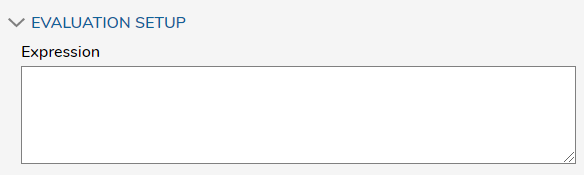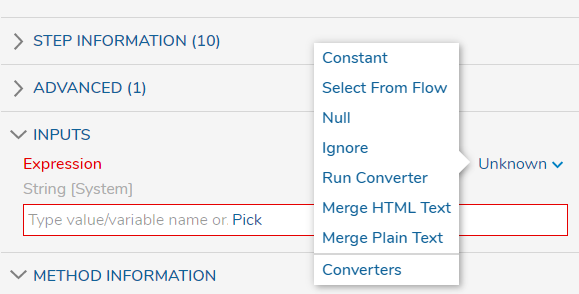## Expression List

The chart below is a list of expressions that can be used in the Evaluate Expression and Evaluate Dynamic Expression steps to perform NCalc Functions. NCalc functions populate this list.

For additional information on NCalc expressions, see: NCalc.
NAMEDESCRIPTIONUSAGERESULT
AbsReturns the absolute value of a specified number.Abs(-1)1M
AcosReturns the angle whose cosine is the specified number.Acos(1)0d
AsinReturns the angle whose sine is the specified number.Asin(000d
AtanReturns the angle whose tangent is the specified number.Atan(0)0d
CeilingReturns the smallest integer greater than or equal to the specified number.Ceiling(1.5)2d
CosReturns the cosine of the specified angle.Cos(0)1d
ExpReturns e raised to the specified power.Exp(0)1d
FloorReturns the largest integer less than or equal to the specified number.Floor(1.5)1d
IEEERemainderReturns the remainder resulting from the division of a specified number by another specified number.IEEERemainder(3, 2)-1d
LogReturns the logarithm of a specified number.Log(1, 10)0d
Log10Returns the base 10 logarithm of a specified number.Log10(1)0d
MaxReturns the larger of two specified numbers.Max(1, 2)2
MinReturns the smaller of two numbers.Min(1, 2)1
PowReturns a specified number raised to the specified power.Pow(3, 2)9d
SignReturns a value indicating the sign of a number.Sign(-10)-1
SinReturns the sine of the specified angle.Sin(0)0d
SqrtReturns the square root of a specified number.Sqrt(4)2d
TanReturns the tangent of the specified angle.Tan(0)0d
TruncateCalculates the integral part of a number.Truncate(1.7)1
InReturns whether an element is in a set of values.in(1 + 1, 1, 2, 3)true
IfReturns a value based on a condition.if(3 % 2 = 1, 'value is true', 'value is false')'value is true'

## Example A: Evaluate Expression

This example takes in user-defined values for each test value and averages them out through use of the Evaluate Expression step.

1. From a Designer Project, select the CREATE FLOW button from the top Action bar. Select CREATE, Flow, then provide a Name and click CREATE.
2. Attach an Evaluate Expression step to the Done path of the Start step from DATA > NUMBERS.
3. Set up the Expression by selecting the Evaluate Expression step to navigate to the Properties tab for the step.
1. Under EVALUATION SETUP > Expression input "(x+y+z)/3"
This configuration will evaluate the sum of three variables, and divide that sum by three.
2. Connect the Done path of this step to the End step.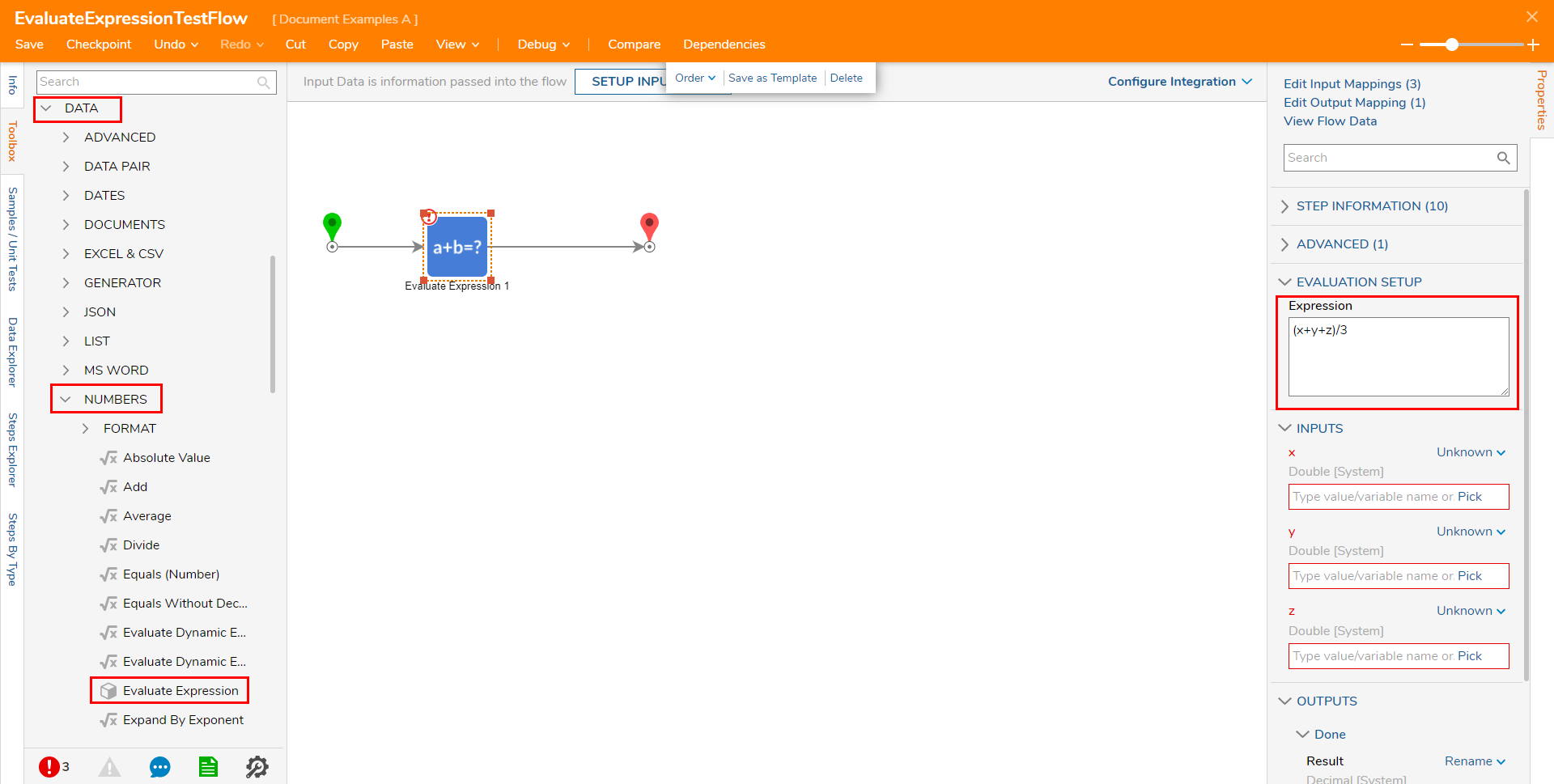4. Place a Show Form step from Toolbox > FAVORITE STEPS before the Evaluate Expression step.

5. Select the Show Form step and click the PICK OR CREATE FORM step.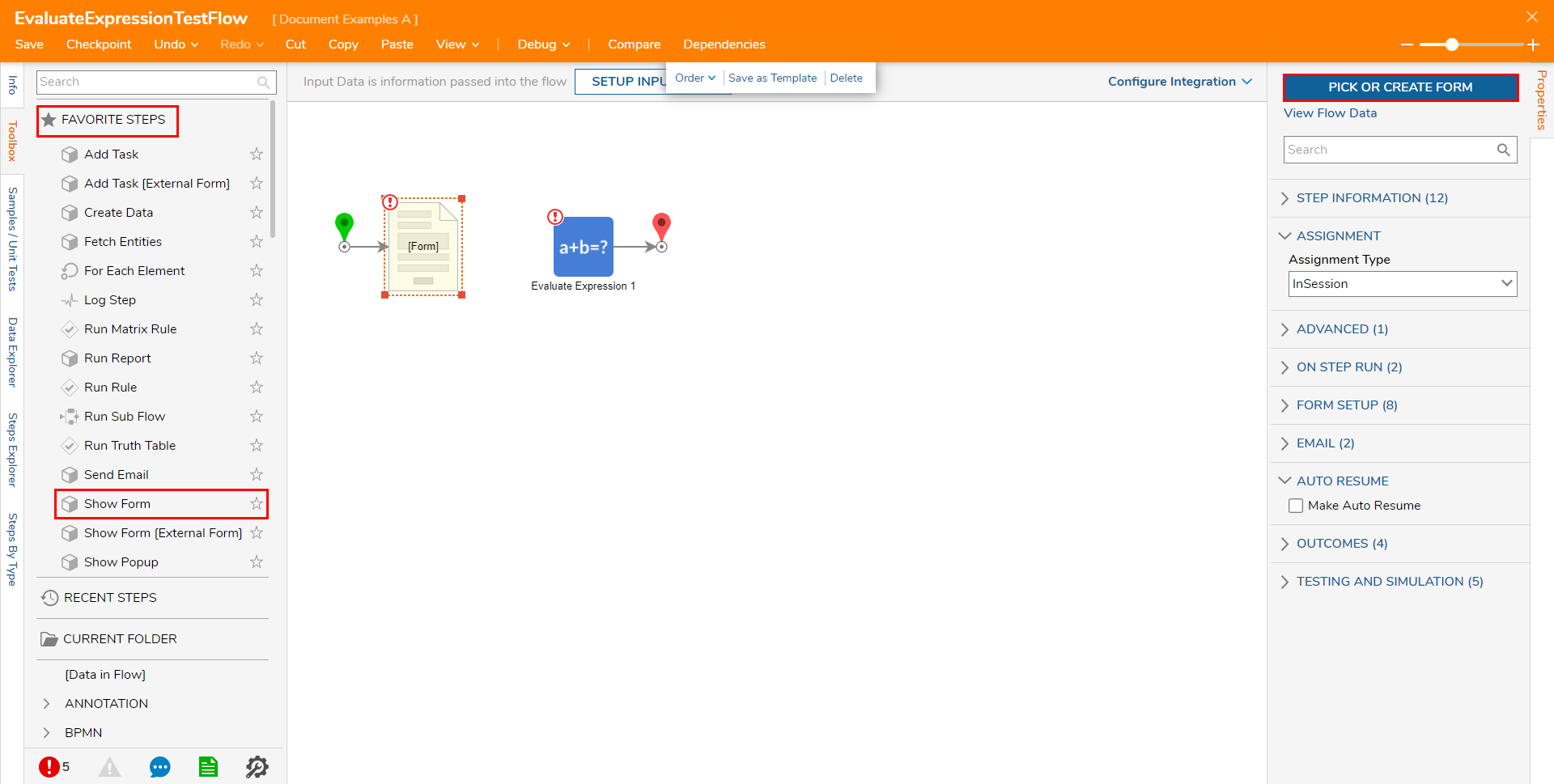1. Click CREATE, choose Form, Name the Form, and then click CREATE to navigate to the Form Designer.

6. Drag in a Button component from Toolbox > FAVORITES; name this Button "Submit".

7. Add three Number Box components.

1. Provide each one with a name that corresponds to each Exam (First Exam, Midterm, Final Exam).

While adding each Number Box component and naming them, press Shift+Ctl+Enter on the keyboard to add a label to the left of that component.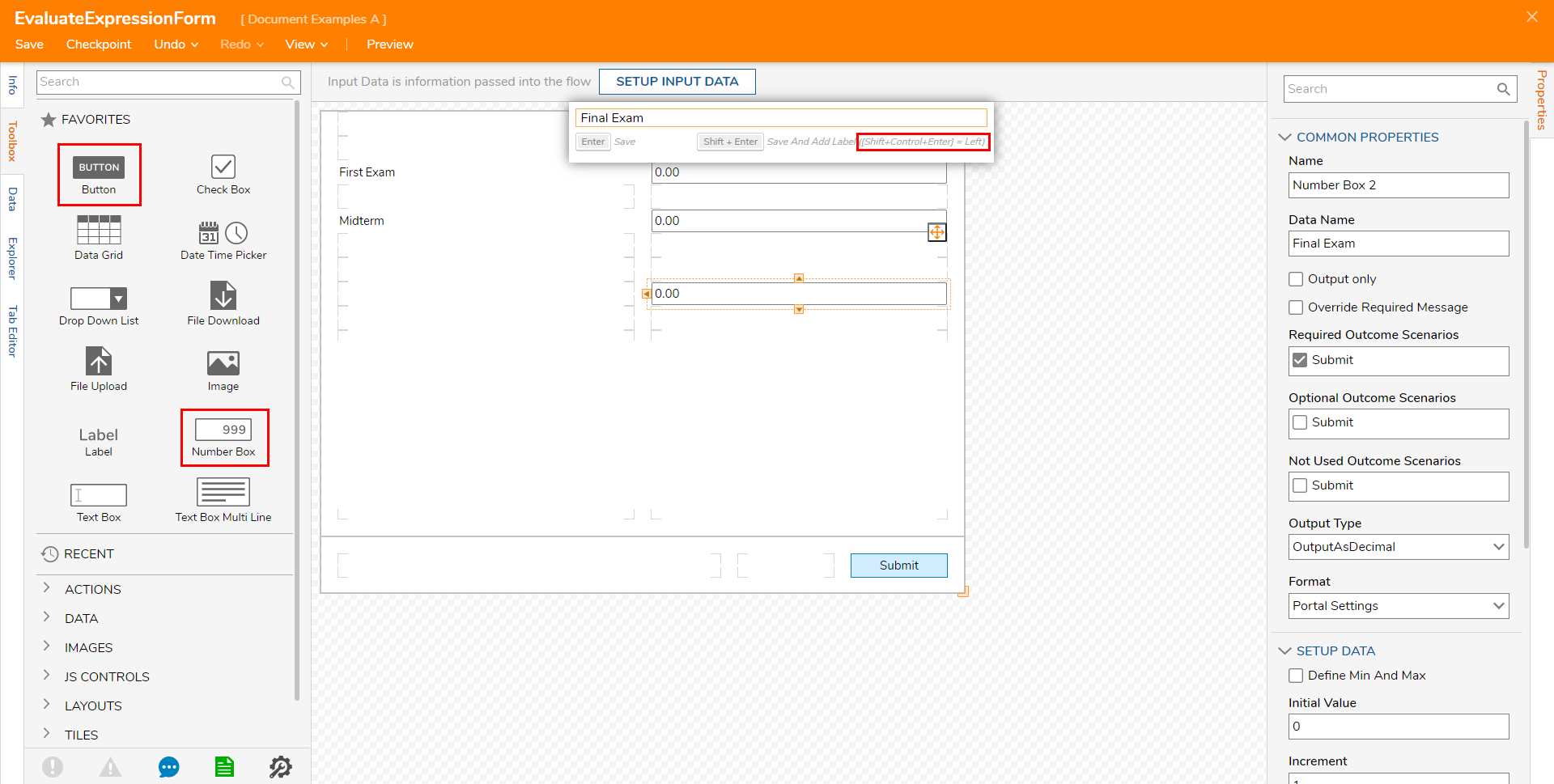8. Save and close the Form Designer to the return to the Flow Designer.

9. Select the Show Form step and set each item under INPUTS' mapping to Ignore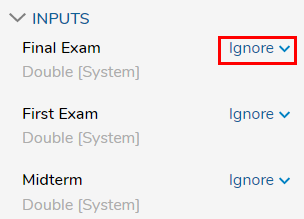10. Connect the Show Form step to the Evaluate Expression step.

11. Select the Evaluate Expression step and map each Output from the Form step to its respective value under the Evaluate Expression's INPUTS.

First Exam to X, Midterm to Y, and Final Exam to Z.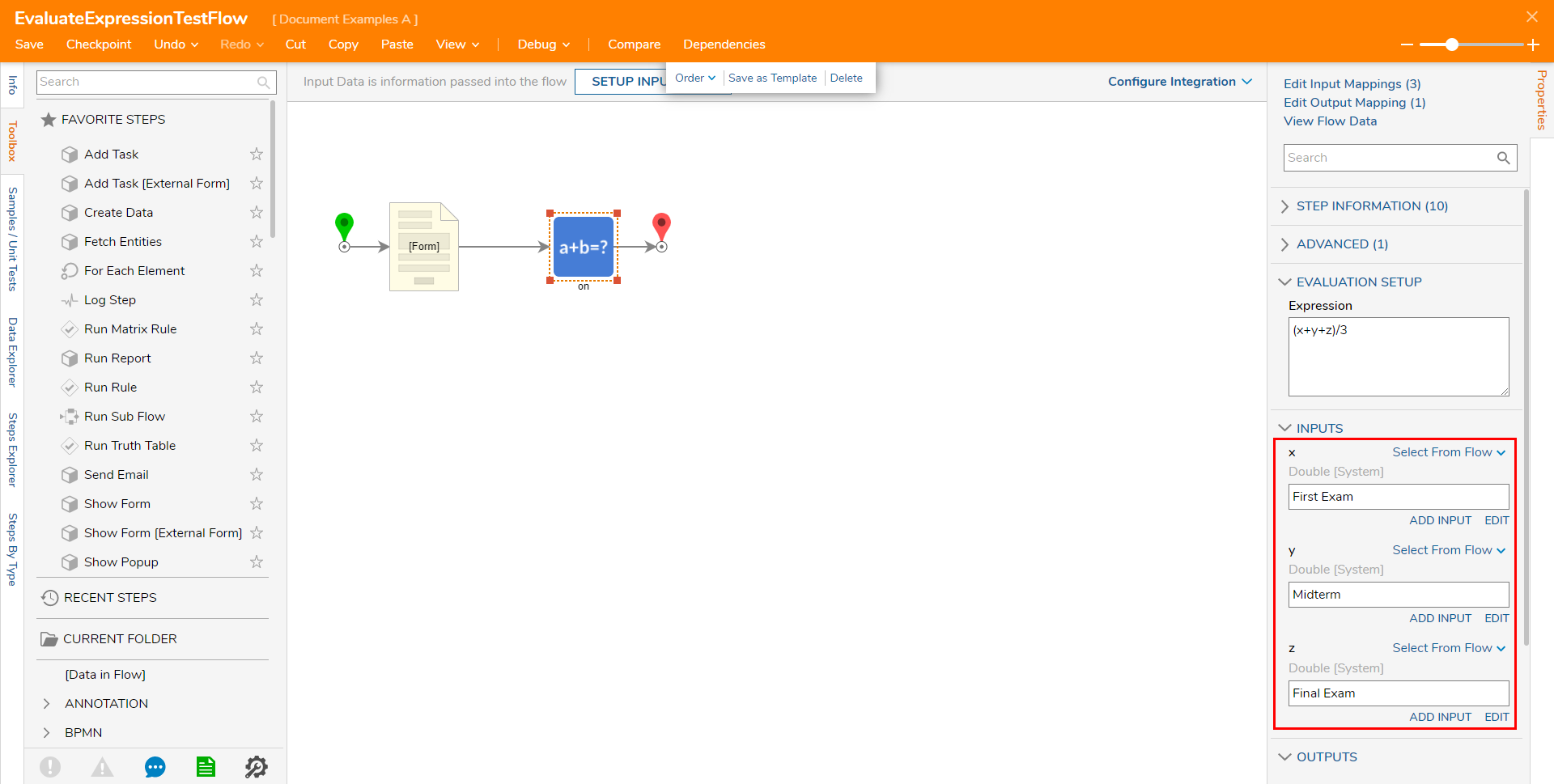12. Save the Flow, then close if desired.

## Debug

1. From the Flow Designer, select the Debug link.
2. Click the START DEBUGGING button.
3. Enter a value for each test score in the Number Boxes, then click Submit.
The Flow will continue running to the Evaluate Expression step, where the average of the three scores will be calculated.
4. Click the Evaluate Expression step and select Execution 1 > View Input/Output Data to view the three scores and the calculated average.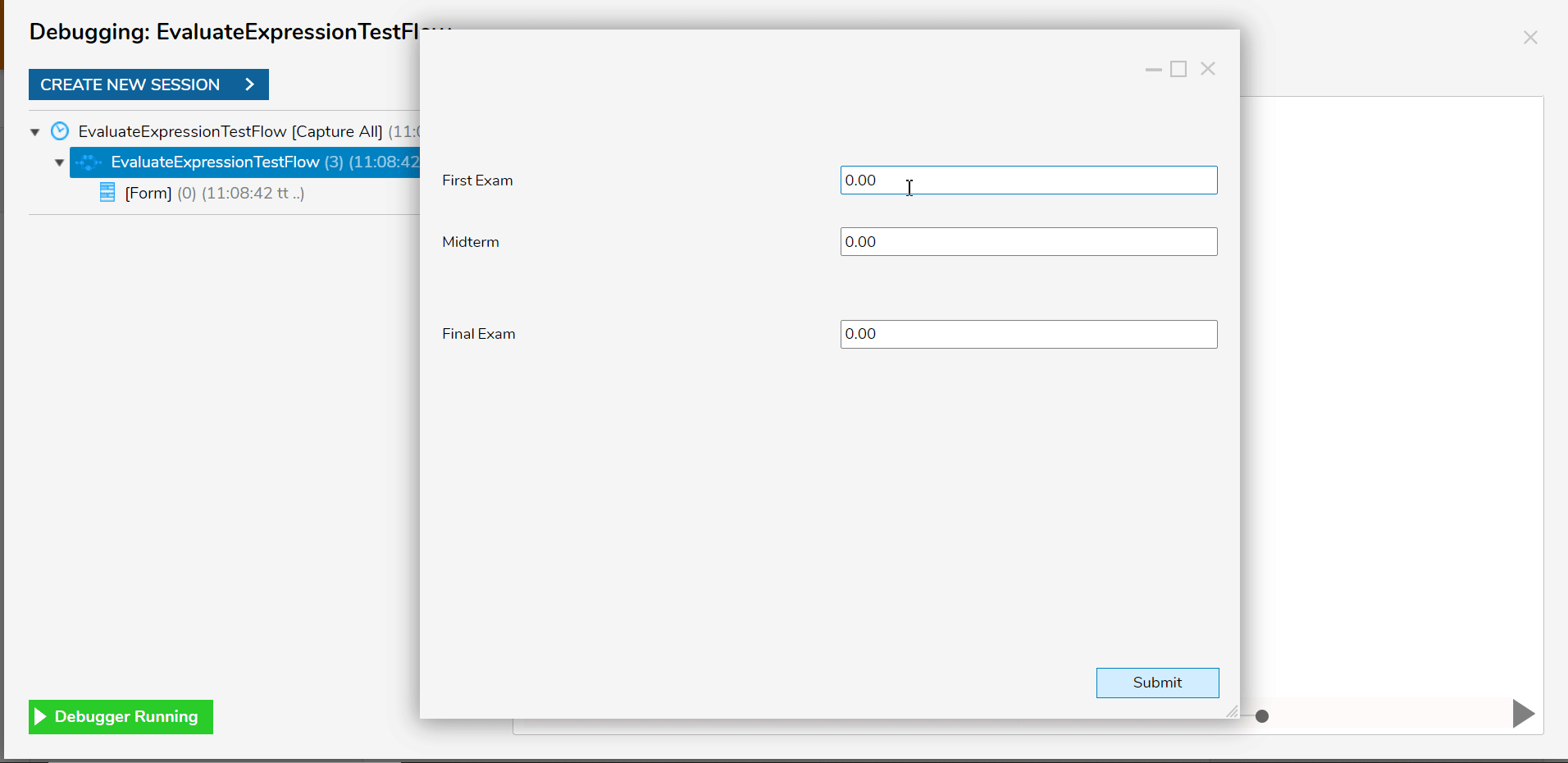## Example B: Evaluate Dynamic Expression

The following example demonstrates how to use a Create Data with an Evaluate Dynamic Expression step to solve an algebraic expression.

1. From a Designer Project, select CREATE FLOW from the Global Action Bar, and CREATE a new Flow
2. From the Flow Designer, attach a Create Data step from the FAVORITE STEPS category of the Toolbox tab, to the Start step.
3. From the Properties tab of the Create Data step, under DATA > Data to Create, and select the SHOW EDITOR link.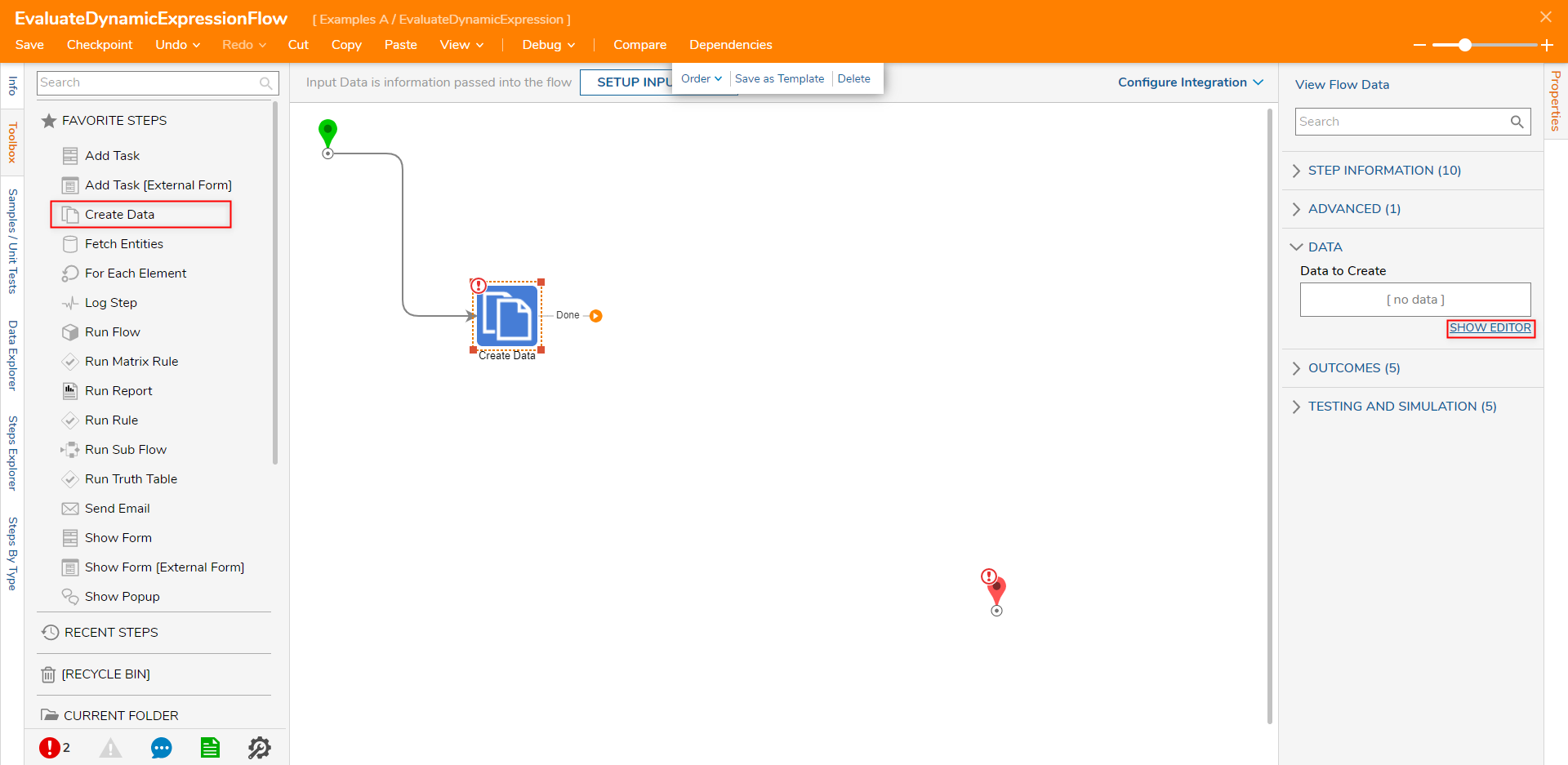4. From the Data to Create screen, NAME the data "Expression" and select String from the TYPE dropdown. Under INPUT, by utilizing NCalc expressions (see the chart above), map an algebraic expression. Then click Save and close via X.
Mapping an Expression
When defining an algebraic expression, NCalc expressions can be used in a similar manner to how they are typically used on a scientific or graphing calculator.

For this example, define the Expression as "(Sqrt(2+2))*10". In this case, the utilized NCalc expression, "Sqrt" is used to calculate the square root of the value in the parentheses.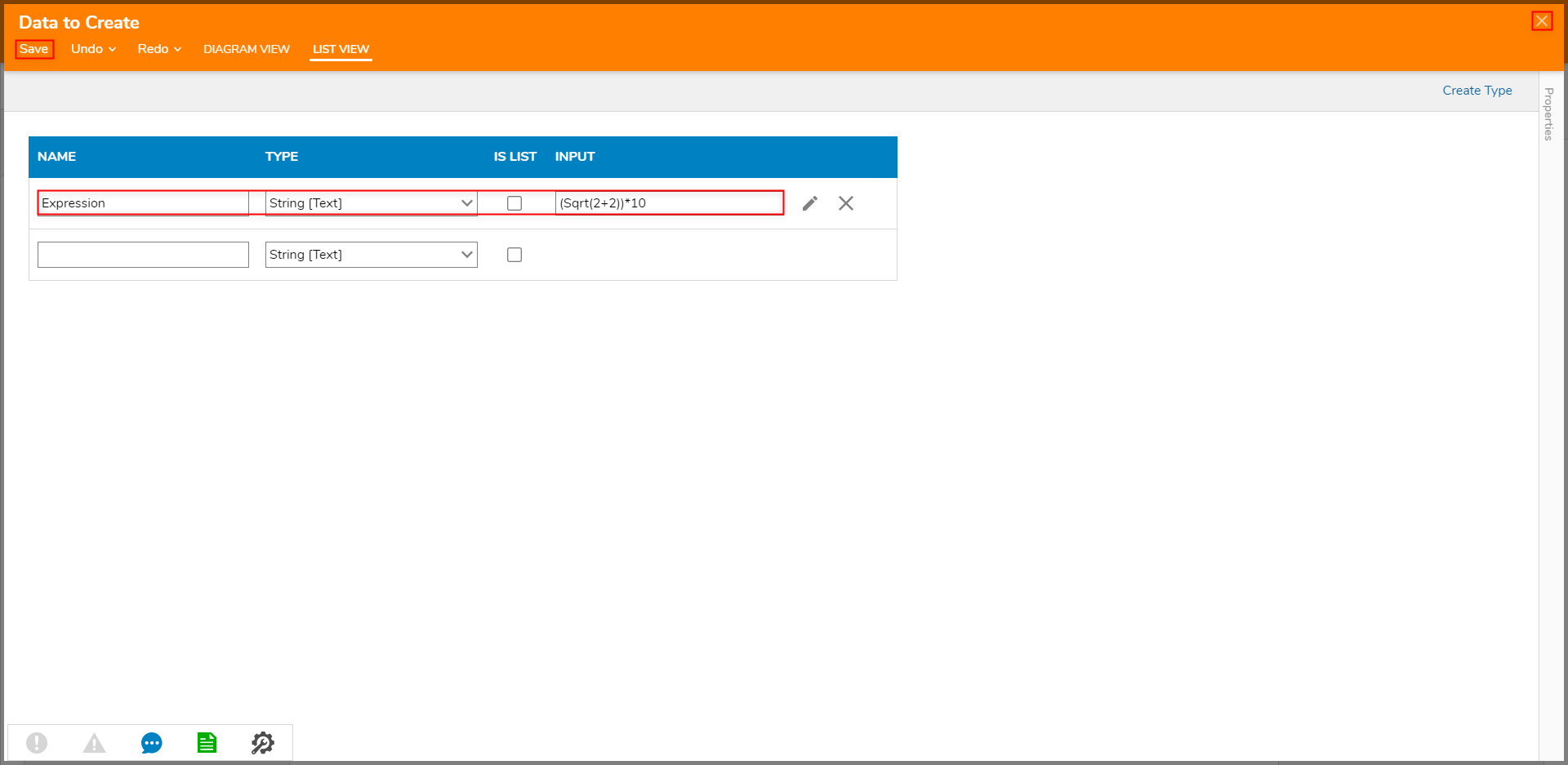5. From Toolbox > DATA > NUMBERS, attach an Evaluate Dynamic Expression step to the Done path of the Create Data step. Then connect the Done path of the Evaluate Dynamic Expression step to the End step.

6. From the Evaluate Dynamic Expression step's Properties, Select From Flow map Expression to its respective INPUT.

By using an Evaluate Dynamic Expression step, users have the option to source the Expression Input in multiple dynamic ways. These ways include, Constant, Null, Ignore, Run Converter, Merge HTML Text, and Merge Plain Text options. This differs from being limited to only being able to define the Expression via Constant mapping.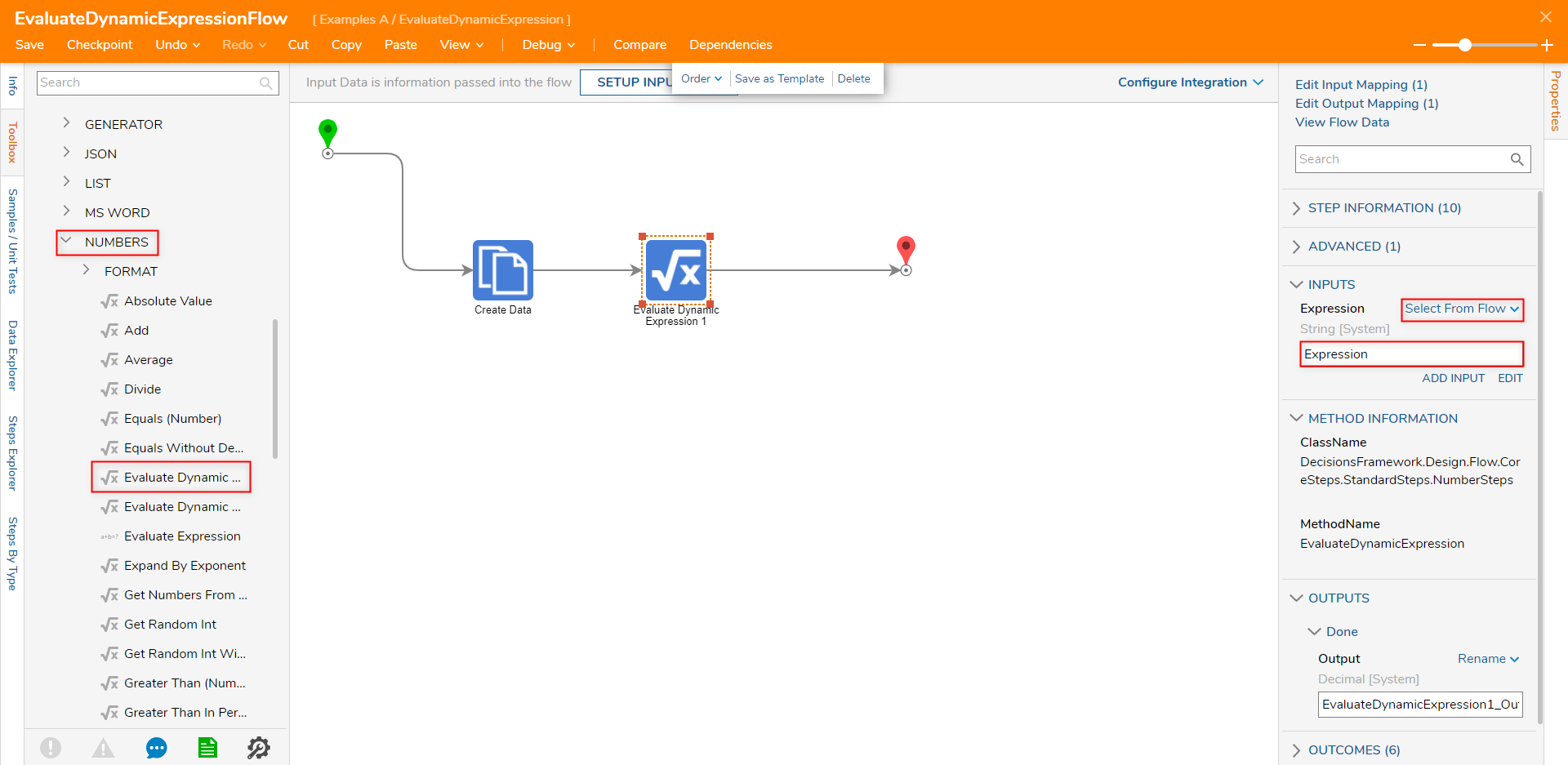7. Save the Flow, then if desired, close via X.

## Debug

1. From the Flow Designer, select the Debug link from the top Action bar. Click START DEBUGGING.
Expression Evaluation
Upon running the Flow, the Evaluate Dynamic Expression step calculates the outcome of the Dynamic Expression. The step accomplishes this by checking any evaluating any utilized NCalc expressions and solving the equation via standard algebraic order of operations.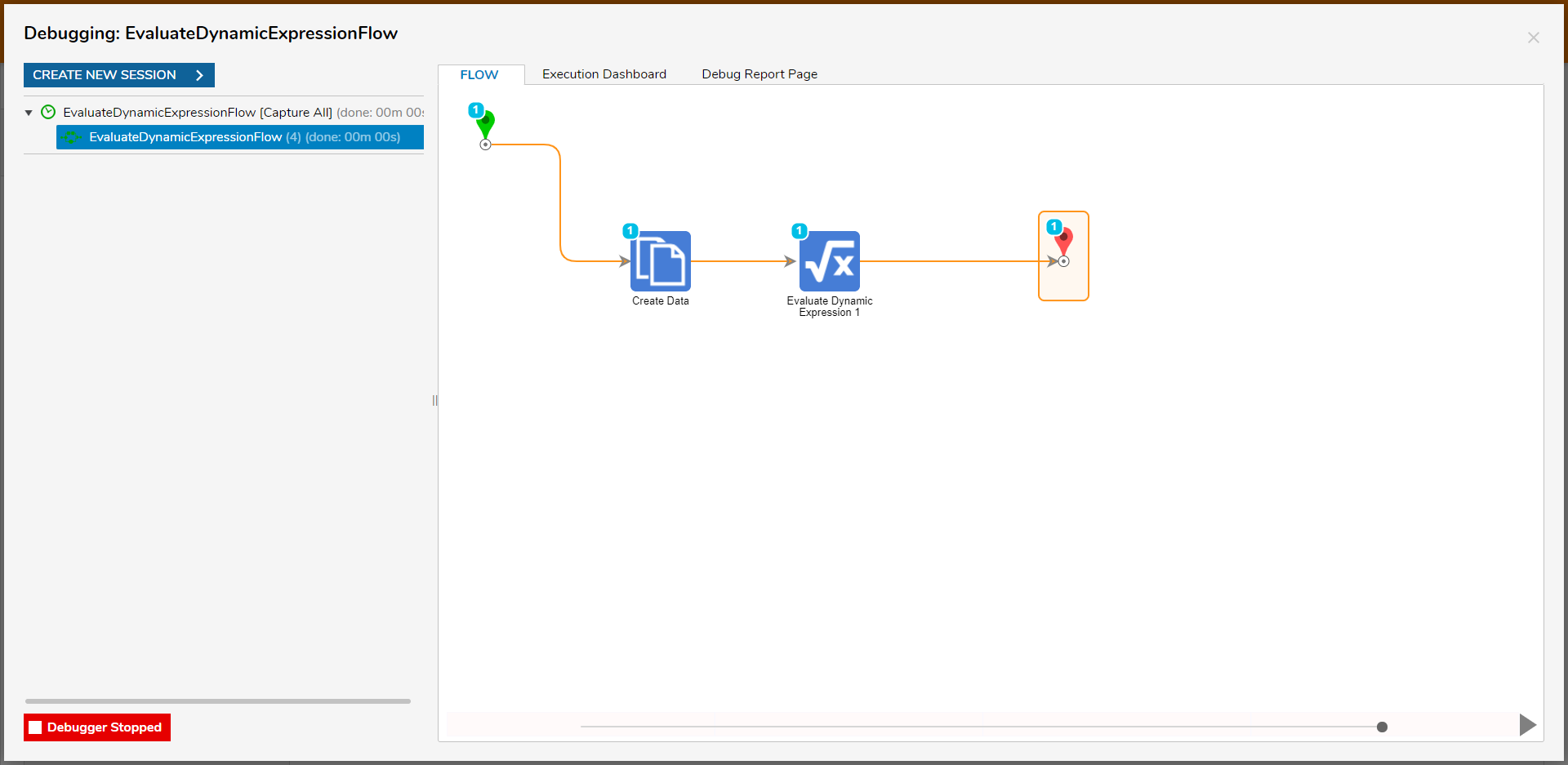2. After the Flow runs, select Evaluate Dynamic Expression 1 > Execution 1 > View Input/Output; verify that the step properly solved the Expression.